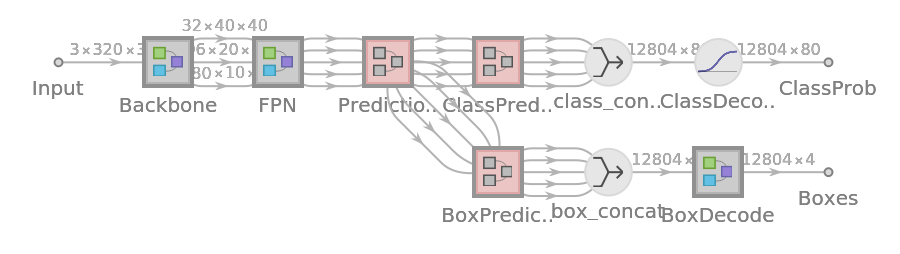# SSD Feature Pyramid Nets Trained onMS-COCO Data

Detect and localize objects in an image

This family of object detection models introduces a feature pyramid network (FPN) to effectively recognize objects at different scales. To make lower-level feature maps semantically stronger, FPN employ top-down pathways to upsample higher resolution features, which are then merged with features from bottom-up pathway via lateral connections. All models inside the object detection family have separate modules for box and class predictions except for MobileNetV2 models, which share some computations before making the final predictions. In addition, all models had been trained using "Focal Loss," which addresses the imbalance between foreground and background classes that arises within single-stage detectors.

## Training Set Information

• Microsoft COCO, a dataset for image recognition, segmentation and captioning, consisting of more than three hundred thousand images overall consisting of 80 object classes.

## Model Information

•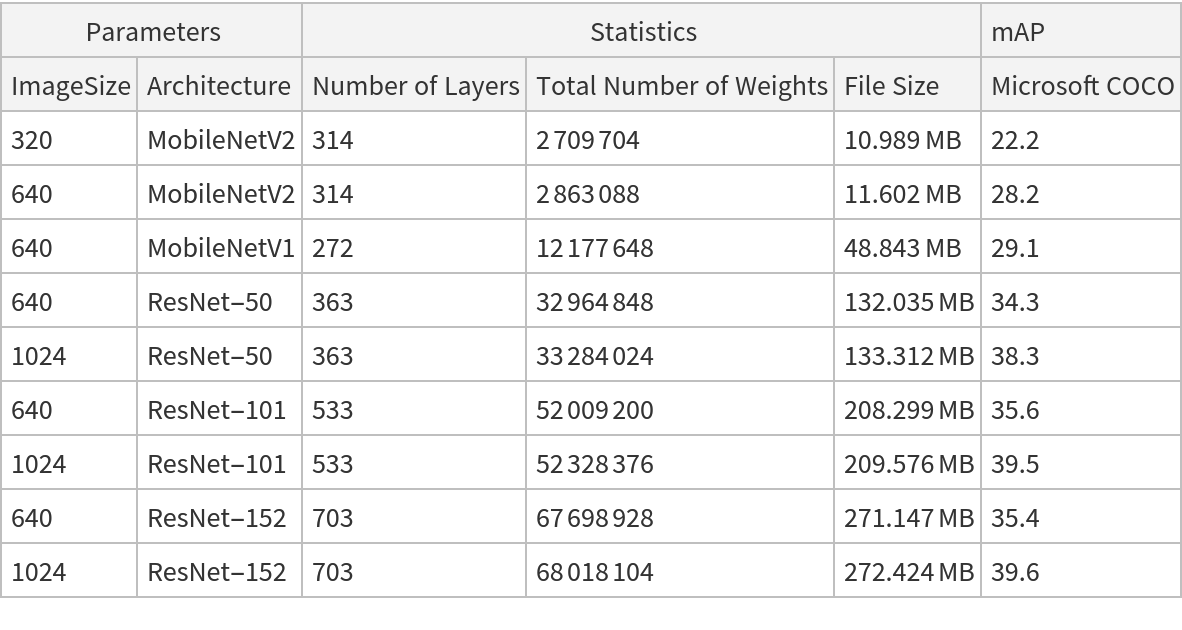## Examples

### Resource retrieval

Get the pre-trained net:

 In:=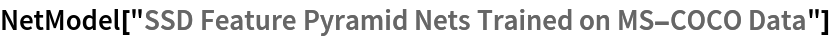Out=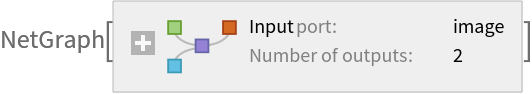### NetModel parameters

This model consists of a family of individual nets, each identified by a specific parameter combination. Inspect the available parameters:

 In:=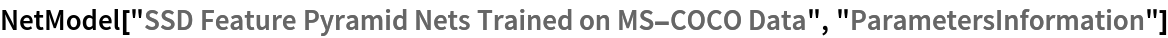Out=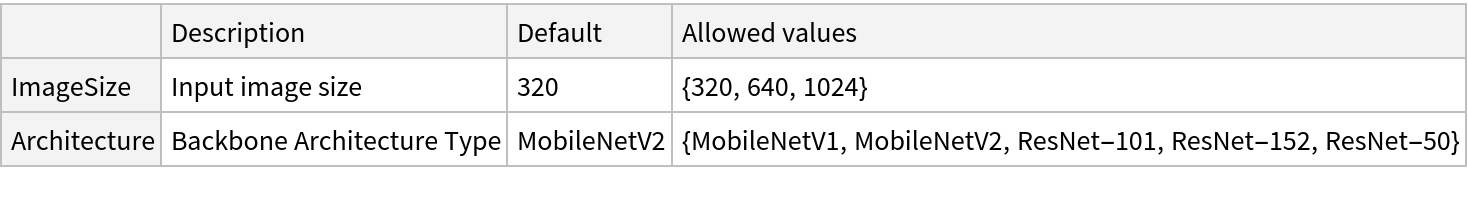Pick a non-default net by specifying the parameters:

 In:=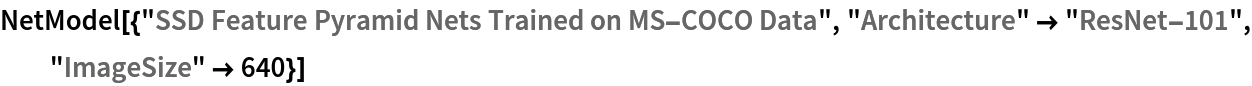Out=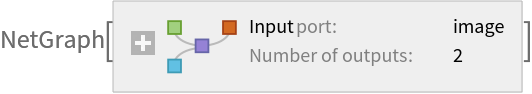Pick a non-default uninitialized net:

 In:=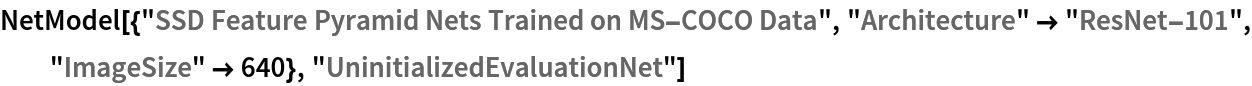Out=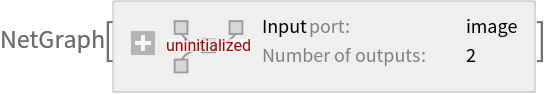### Evaluation function

Define the label list for this model:

 In:=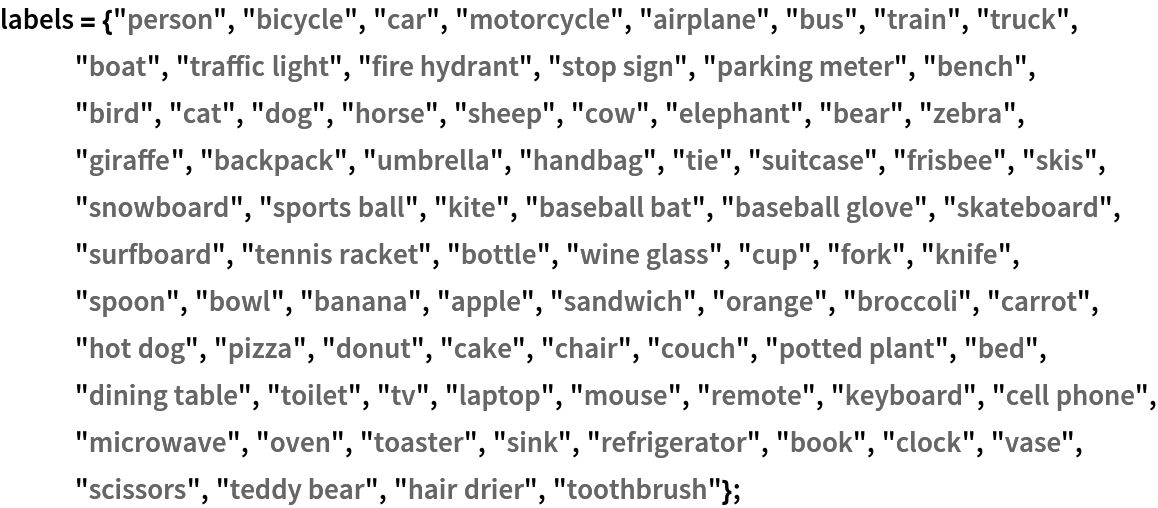Write an evaluation function to scale the result to the input image size and suppress the least probable detections:

 In:=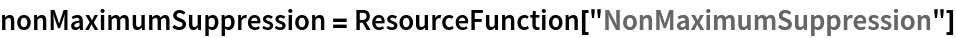Out=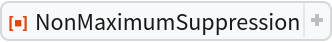In:=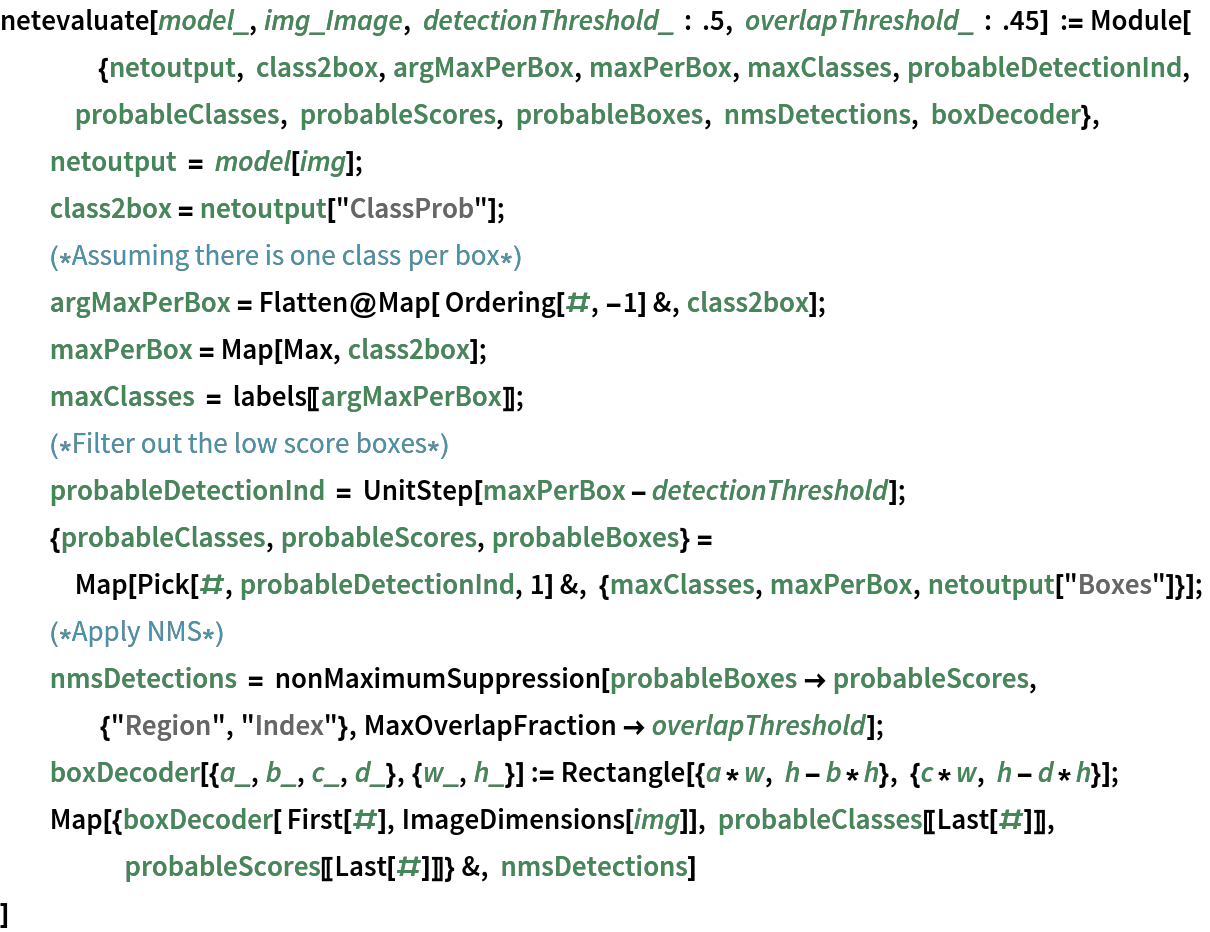### Basic usage

Obtain the detected bounding boxes with their corresponding classes and confidences for a given image:

 In:=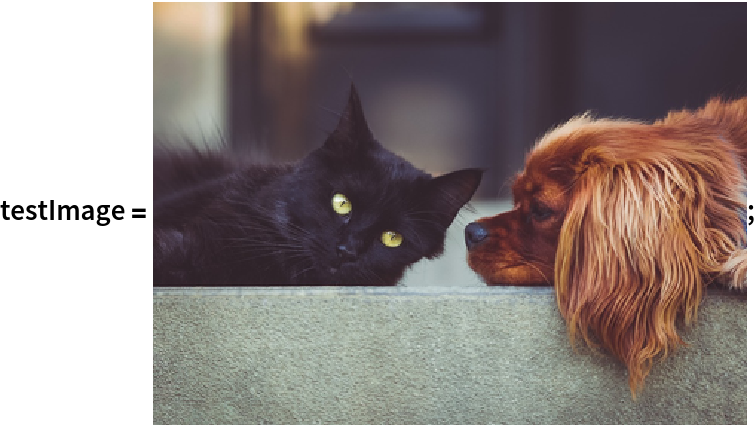In:=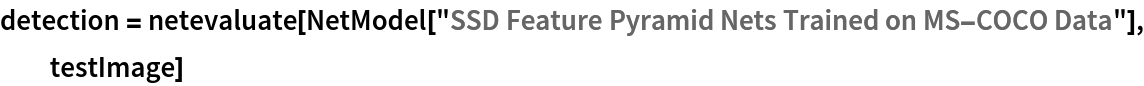Out=Inspect which classes are detected:

 In:=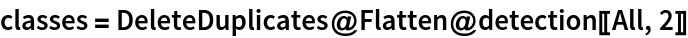Out=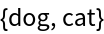Visualize the detection:

 In:=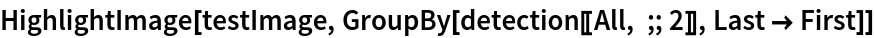Out=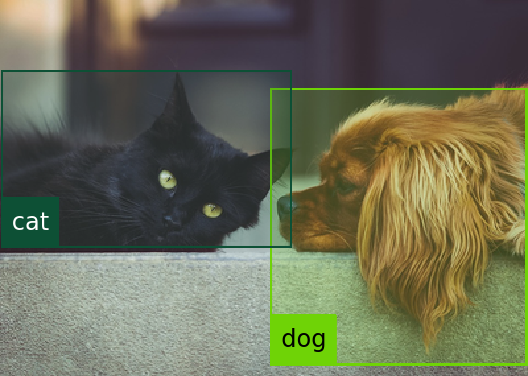### Network result

The network computes 12,804 bounding boxes and the probability that the objects in each box are of any given class:

 In:=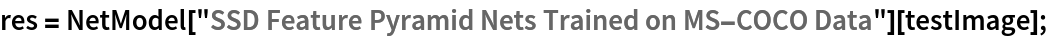In:=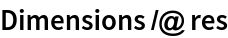Out=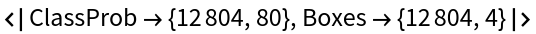Change coordinate system into a graphics domain:

 In:=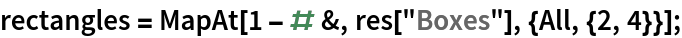Visualize all the boxes predicted by the net scaled by their "objectness" measures:

 In:=In:=Out=Visualize all the boxes scaled by the probability that they contain a cat:

 In:=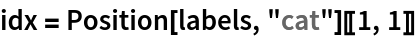Out=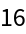In:=Out=Superimpose the cat prediction on top of the scaled input received by the net:

 In:=Out=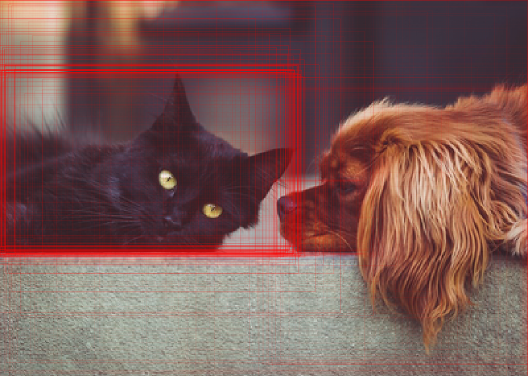### Net information

Inspect the number of parameters of all arrays in the net:

 In:=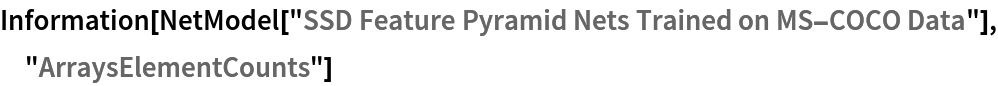Out=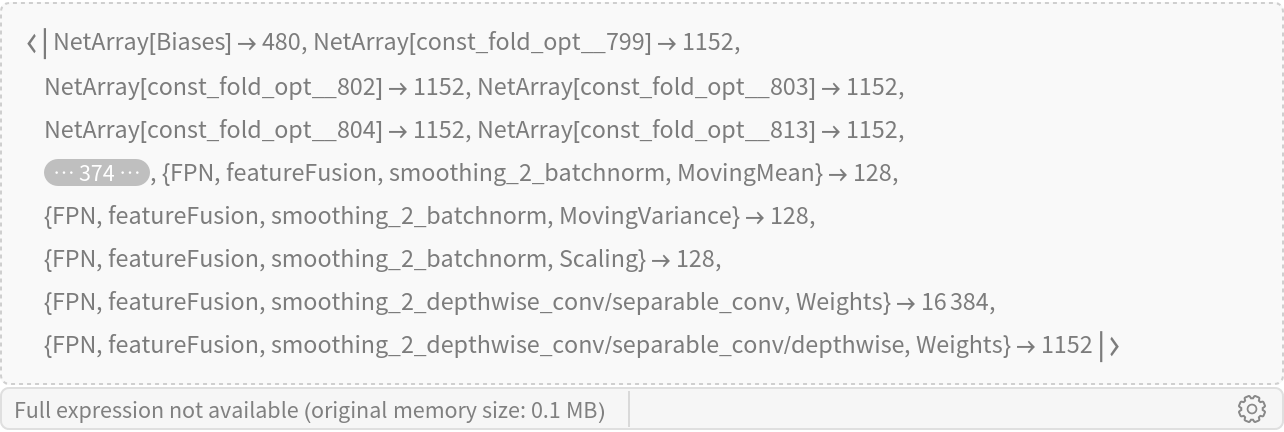Obtain the total number of parameters:

 In:=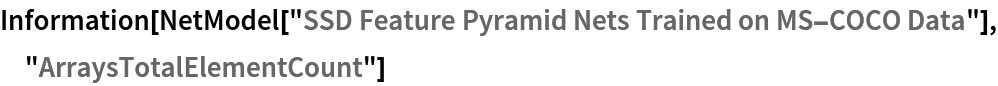Out=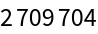Obtain the layer type counts:

 In:=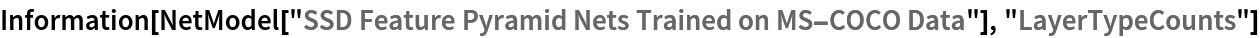Out=Display the summary graphic:

 In:=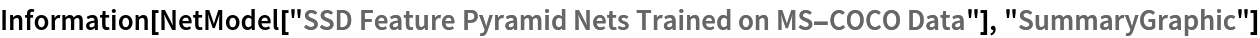Out=